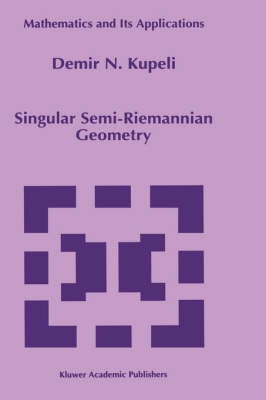•# Singular Semi-Riemannian Geometry - Mathematics and Its Applications 366 (Hardback)

(author)
£109.99
Hardback 181 Pages / Published: 30/04/1996
• We can order this from the publisher

Usually dispatched within 15 working days

This book is an exposition of "Singular Semi-Riemannian Geometry"- the study of a smooth manifold furnished with a degenerate (singular) metric tensor of arbitrary signature. The main topic of interest is those cases where the metric tensor is assumed to be nondegenerate. In the literature, manifolds with degenerate metric tensors have been studied extrinsically as degenerate submanifolds of semi Riemannian manifolds. One major aspect of this book is first to study the intrinsic structure of a manifold with a degenerate metric tensor and then to study it extrinsically by considering it as a degenerate submanifold of a semi-Riemannian manifold. This book is divided into three parts. Part I deals with singular semi Riemannian manifolds in four chapters. In Chapter I, the linear algebra of indefinite real inner product spaces is reviewed. In general, properties of certain geometric tensor fields are obtained purely from the algebraic point of view without referring to their geometric origin. Chapter II is devoted to a review of covariant derivative operators in real vector bundles. Chapter III is the main part of this book where, intrinsically, the Koszul connection is introduced and its curvature identities are obtained. In Chapter IV, an application of Chapter III is made to degenerate submanifolds of semi-Riemannian manifolds and Gauss, Codazzi and Ricci equations are obtained. Part II deals with singular Kahler manifolds in four chapters parallel to Part I.

Publisher: Springer
ISBN: 9780792339960
Number of pages: 181
Weight: 1000 g
Dimensions: 235 x 155 x 12 mm
Edition: 1996 ed.